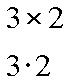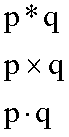# Expression Evaluation, A Few Notes About Multiplication

We have used an asterisk for the multiplication operator. This is not really unusual; many computer languages and graphics calculators work this way. So, a statement such as this can mean three times two:

3 * 2

But there are other common ways to note multiplication. Each of the following are usually accepted to mean three times two:If you were using variables, such as p and q, multiplication could be shown in any of these ways:In fact, in such cases involving variables you can indicate a multiplication by simply placing the variables next to each other. The following means p times q:

pq

The above method does not work for numbers, though. If you try to state three times two using this method you end up with:

32

Which, obviously, is thirty-two, not at all equal to six. If you want to put two numbers next to each other in order to indicate multiplication, you need to use parentheses. Any of the following correctly states three times two:

3(2)

(3)2

(3)(2)

And what if you want to show a number times a variable? Well, here is five times x:

5x

The number comes first, and no parentheses are required. Although strictly not incorrect, the following forms are not normally used in mathematics notation:

x5

(5)x

5(x)

(5)(x)

Where are you?

Here: Just a Few Notes about Multiplication

Custom Search